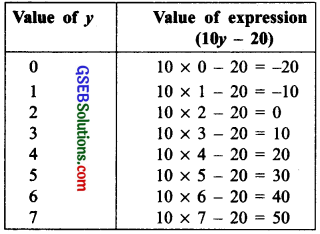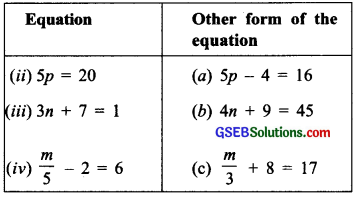# GSEB Solutions Class 7 Maths Chapter 4 Simple Equations InText Questions

Gujarat Board GSEB Textbook Solutions Class 7 Maths Chapter 4 Simple Equations InText Questions and Answers.

## Gujarat Board Textbook Solutions Class 7 Maths Chapter 4 Simple Equations InText QuestionsTry These (Page 78)

Question 1.
The value of the expression (10y – 20) depends on the value of y. Verify this by giving five different values to y and finding for each y the value of (10y – 20). From the different values of (10y – 20) you obtain, do you see a solution to 10y – 20 = 50? If there is no solution, try giving more values to y and find whether the condition 10y – 20 = 50 is met.
Solution:Thus, the condition 10y – 20 is true for y = 7.

Try These (Page 80)

Question 1.
Write atleast one other form for each equation (ii), (iii) and (iv) of Example 2 on Page 80.
Solution:
Statement of other form of the equation:(a) Taking away 4 from five times p gives 16.
(b) Add 9 to four times n to get 45.
(c) Add 8 to one-third of m to get 17.Try These (Page 88)

Question 1.
Start with the same step x = 5 and make two different equations. Ask two of your classmates to solve the equations. Check whether they get the solution x = 5.
Solution:
I. x = 5
Multiplying both sides by 2, we have
2x = 10
Adding 6 to both sides, we have
or 2x + 6 = 10 + 6
or 2x + 6 = 16 is an equation.

II. x = 5
Dividing both sides by 3, we have
$$\frac { x }{ 3 }$$ = $$\frac { 5 }{ 3 }$$
Subtracting 2 from both sides, we have
$$\frac { x }{ 3 }$$ – 2 = $$\frac { 5 }{ 3 }$$
or $$\frac { x }{ 3 }$$ – 2 = $$\frac { 5-6 }{ 3 }$$ = $$\frac { -1 }{ 3 }$$
Thus, $$\frac { x }{ 3 }$$ – 2 = $$\frac { -1 }{ 3 }$$ is an equation. Solution to I
2x + 6 = 16
Subtracting 6 from both sides, we have
2x + 6 – 6 = 16 – 6
or 2x = 10
Dividing both sides by 2, we have
$$\frac { 2x }{ 2 }$$ = $$\frac { 10 }{ 2 }$$
Solution to II
$$\frac { x }{ 3 }$$ – 2 = $$\frac { – 1 }{ 3 }$$
Adding 2 to both sides, we have
$$\frac { x }{ 3 }$$ – 2 + 2 = $$\frac { -1 }{ 3 }$$ + 2 or $$\frac { x }{ 3 }$$ = $$\frac { 5 }{ 3 }$$
Multiplying both sides by 3, we have
$$\frac { x }{ 3 }$$ x 3 = $$\frac { 5 }{ 3 }$$ x 3 or x = 5Try These (Page 88)

Question 1.
Try to make two number puzzles, one with the solution 11 and another with 100.
Solution:
I. A puzzle having the solution as 11:
Think of a number. Multiply it by 3 and add 7. Tell me the sum.
If the sum is 40, then the number is 11.

II. A puzzle having the solution as 100:
Think of a number. Divide it by 4 and add 5. Tell me what you get.
If you get 30, then the number is 100.

Note: Instead of making the same operation on both sides, we can move a number from one side to another by changing its sign from (+) to (-) and (-) to (+). This is called ‘transposing a number’. Thus, transposing a number is the same as adding or subtracting the number from both sides. In doing so, the sign of the number has to be changed.

Try These (Page 90)

Question 1.
(i) When you multiply a number by 6 and subtract 5 from the product, you get 7. Can you tell what the number is?
(ii) What is that number one-third of which added to 5 gives 8?
Solution:
(i) Let the number be x.
∴ Multiply the number by 6, we have 6x. Now, according to the condition, we have
6x – 5 = 7
Transposing 5 from L.H.S. to R.H.S., we have
6x = 7 + 5 = 12
Dividing both sides by 6, we have
$$\frac { 6x }{ 6 }$$ = $$\frac { 12 }{ 6 }$$
or x = 2
∴ The required number = 2

(ii) Let the required number be x.
∵ One-third of the number = $$\frac { 1 }{ 3 }$$x
∴ According to the condition, we have
5 + $$\frac { 1 }{ 3 }$$x = 8
Transposing 5 from L.H.S. to R.H.S., we have 1
$$\frac { 1 }{ 3 }$$x = 8 – 5 = 3
Multiplying both sides by 3, we have
3 x $$\frac { 1 }{ 3 }$$ = 3 x 3
or x = 9
Thus, the required number = 9.Try These (Page 90)

Question 1.
There are two types of boxes containing mangoes. Each box of the larger type contains 4 more mangoes than the number of mangoes contained in 8 boxes of the smaller type. Each larger box contains 100 mangoes. Find the number of mangoes contained in the smaller box?
Solution:
Let the number of mangoes contained in the smaller box be x.
∴ Number of mangoes in 8 smaller boxes = 8x Now, according to the condition, we have 8x + 4 = 100
Transposing 4 to R.H.S., we have
8x = 100 – 4
or 8x = 96
Dividing both sides by 8, we have
$$\frac { 8x }{ 8 }$$ = $$\frac { 96 }{ 8 }$$
or x = 12
Thus, the number of mangoes in the smaller box = 12.